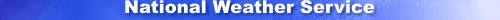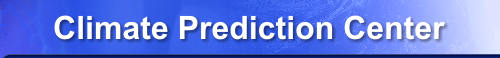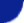Site Map News Organization SearchHOME > Monitoring_and_Data > Oceanic and Atmospheric Data > Reanalysis: Atmospheric Data > wgrib2-ens_processing

### Introduction (wgrib2 v2.0.8+)

The -ens_processing option has several problems in wgrib2 v2.0.8. The number of threads should be set to one on newer gcc compilers, 75% is inaccuracte, precip trace amounts (cpc private) is bad, and a possible seg fault could occur. (The out-of-bounds array value is multipled by zero, so it doesn't affect the calculation.)

The -ens_processing option takes the ensemble member data and creates a pre-defined set of ensemble statistics. If you want find the probability that a certain field has values exceeding a limit, you should look at trick 65 in the wgrib2 tricks page.

The -ens_processing option is used to create various ensemble statistics such as minimum, maximum, average, spread, and various percentiles in order to describe the PDF. The values are calculated with respect to all the ensemble members. The calculations are different from the calculations used by the operational ensemble forecasts done at NCEP. So fields like the various percentiles and spread will have different values from the NCEP operational products. The results from the -ens_processing option should not be considered to be replacements for the official products because of the different algorithms used. (The exception will be the future climate reanalysis produced by CPC/NCEP.)

The percentiles values were chosen because the future CPC/NCEP climate reanalysis (CORe) will be using 80 ensemble members.

The calculated variables are

```1) ensemble mean,  em = sum(x(i))/n,  i=1..n
2) ensemble spread, RMSE = sqrt(sum((x(i)-em)**2)/n)  note: n is used rather than n-1
3) minimum = minimum value over all ensemble members (for each grid point)
4) maximum = maximum value over all ensemble members (for each grid point)
5) 10 percentile
6) 25 percentile
7) 50 percentile (median)
8) 75 percentile
9) 90 percentile

Note: these calculations done when all the ensemble members have
values.  This will affect parameters like the cloud-top temperature
when some of the ensemble members are cloud free and have no cloud-
top temperatures. The -enq_qc calculates the ensemble mean and spread
ignoring the undefined values.

There are a few common ways to compute the percentile, wgrib2 uses a method
recommended by NIST:

https://www.itl.nist.gov/div898/handbook/prc/section2/prc262.htm

percentile: sort values (1..N), p=percentile/100
x(1) if p ≤ 1/(N+1)
x(p*(N+1)) if 1/(N+1) ≤ p &le N/(N+1)
x(N) if N/(N+1) ≤ p ≤ 1

x(r) = x(floor(r))(1-(r-floor(r)) + x(floor(r)+1)(r-floor(r))
for non-integral r

For the probabilities, a count is used to determine them.

As previously mentioned, the above calculations may differ from those used
in deriving the operational products.
```

The -ens_processing option was developed for the future CORe reanalysis. For this reanalysis, additional fields are enabled by the second parameter set to "1". However, users are warned that these fields are expected to change. Generation of additional fields can be enabled by setting the second parameter to special values.

```10) probability of precipitation > 0 *
11) probability of more than a trace of precipitation (trace=TBD) *
12) probability of 2m temperature greater than 273.15K *
13) 95 percentile for WIND at 10m above ground *

* optional, the definition of trace of precipitation is ad hoc
trace can mean precipitation that will get the rain gauge wet but register as zero
trace can mean less than 0.01 inches
trace can mean less than 0.1 mm
for wgrib2, trace = accumulated precip < 0.xxx mm, or a rate < 0.xxx mm/day.
```

The -ens_processing is unlike most wgrib2 options in that this option can use large amounts of memory. Suppose that you have an 80 member ensemble and are processing the tmp500 field. In order to calculate the percentiles of the tmp500, you need keep all 80 tmp500 fields in memory. As the size of the grid and the number of ensemble member increases, the required memory will increase.

### Code Table 4.3, Type of Generating Process

Grib contains metadata, and one piece is the "generating process". Wgrib2 tries its best to describe how the fields were created. This is documented in detail because if varies between PDT and center that created the grib file.

```Ensemble mean, ensemble spread, ensemble minimum, ensemble maximum:
Code Table 4.3 is preserved from the input file.

Percentiles, Probabilities:
NCEP: code table 4.3 = 199,  Ensemble forecast based on counting
Other centers: code table 4.3 = 4, Ensemble forecast

The NCEP files are more descriptive because a local table is used.
```

### Definition of an Ensemble MEmber

Wgrib2 v2.0.8 defined an ensemble as having the same Product Definition Template (PDT=0, 1, 8, 11) except for perturbation number. The initial time and forecast times could be different as long as the verification time was the same. This allows lagged averge forecast (LAF) ensembles. With wgrib2 v3.0.0, the type of ensemble forecast can be different. (code table 4.6) This means that -ens_processing can now work with a combination of perturbed and control runs.

Limiting the PDTs to 0, 1, 8 and 11 is unnecessarily restrictive. As a result, -ens_processing does not work on aerosols, chemical tracers and simulated satellite data from ensemble members.

### Order of Fields

The -ens_processing requires fields to be in a specific order. The data has to be processed sequentially. If the -ens_processing finds a field that is unlike the previous fields (except for ensemble member id), it writes out the summary fields and starts processing a new variable. The number id of the ensemble members is ignored, and is not even necessary.
```bash-4.1\$ wgrib2 all.grb | head -n 60
1:0:d=2018020600:PRES:mean sea level:84 hour fcst:ENS=+1
2:83628:d=2018020600:PRES:mean sea level:84 hour fcst:ENS=+2
3:164290:d=2018020600:PRES:mean sea level:84 hour fcst:ENS=+3
4:248531:d=2018020600:PRES:mean sea level:84 hour fcst:ENS=+4
..
19:1494083:d=2018020600:PRES:mean sea level:84 hour fcst:ENS=+19
20:1574514:d=2018020600:PRES:mean sea level:84 hour fcst:ENS=+20
21:1657406:d=2018020600:VIS:surface:84 hour fcst:ENS=+1
22:1703817:d=2018020600:VIS:surface:84 hour fcst:ENS=+2
..
39:2488331:d=2018020600:VIS:surface:84 hour fcst:ENS=+19
40:2533546:d=2018020600:VIS:surface:84 hour fcst:ENS=+20
41:2579586:d=2018020600:UGRD:planetary boundary layer:84 hour fcst:ENS=+1
42:2629955:d=2018020600:UGRD:planetary boundary layer:84 hour fcst:ENS=+2
..
```

### Order of Fields: gmerge to the rescue

The -ens_processing requires the fields to be in a specific order (or processed in a specific order). Suppose we have forecasts from 3 ensemble members in files fcst1, fcst2 and fcst3. Now we are going to require that the fields have the same order in these files, and the files have no submessages. Then we can combine these files using gmerge, the resulting file will be in the correct order. (Gmerge has been included with wgrib2 source code for many years.) There is a minor restriction, gmerge doesn't handle grib files which includes non-grib data.

```gmerge output fcst1 fcst2 fcst3
```
The requirements for output to be in the right order.
1. fcstX must have fields in the same order
2. number of forecasts &le 200 for wgrib2 v2.0.8 distribution
3. fcstX must not use submessages
4. the like forecasts must have same PDT except for ensemble number
5. the ensemble number is optional
6. no non-grib data in grib file
You can find gmerge in the wgrib2 public distrbution under the aux_progs directory. If your initial files are not in identical order, you could combine the forecasts and sort the fields so that like fields are adjacent. However, sorting the individual forecasts and then running gmerge would probably be faster.

Now that "output" has the data in the correct order, the option -ens_processing can be used to create the min/max/ave/spread of the ensemble.

```gmerge  output1 fcst1 fcst2 fcst3
wgrib2  output1 -ens_processing output2 x
note: x is a dummy argument, it may be used in the future

For faster processing, we can replace the disk file "output" by a pipe.
This method may or may not work under Windows.

gmerge - fcst1 fcst2 fcst3 | wgrib2 - -ens_processing output x

gmerge - A B C   writes the output to stdout
wgrib2 - (..)    reads the input grib data from stdin

NOTE: must use a recent version of gmerge.  Old versions of gmerge
only accepted a small number of input files and did not allow
output to the pipe by "-".
```

### Fast Processing

Using gmerge, and sending the output to wgrib2 is simple. However, it can be I/O inefficient especially for large ensembles. Consider a small system that has 80 ensemble member data one drive. For the 1st field, the system has to read field-1 of file-1. For the second field, the system has to read field-1 from file-2. For the 81st field, the system has to read field-2 from file-1. Hopefully the disk cache was big enough that the field-2 of file-1 was still cached. The speed of processing depends on the size of disk cache. This example is for a small system, what would happen on a HPC system? Disk cache is much larger but so is the block size. If one job takes a large fraction of the disk cache, the other jobs will be much more disk inefficient.

A better approach is to adopt the technique used in fast averaging. You may be limited by the amount of physical memory on the system.

```cat fcst.2018122600.mem* | wgrib2 - -set_grib_type c3 \
-if (xxx1) -ens_processing out 1 -fi \
-if (xxx2) -ens_proceesing out 1 -fi \
...
-if (xxxN) -ens_proceesing out 1 -fi
```
This approach only has two open files at any one time, and the files are read and written sequentially. This approach is harder to program but is much more I/O friendly for both workstations and HPCs. The drawback is that this approach need to keep all the fields in memory. (#ensemble members x #fields/file x NX x NY x 4 bytes).

On a Cray, the above processing can easily done on a MAMU node. On a compute nodes, you may have to replace the pipe with a temporary file.

### Usage

```-ens_processing FILE Option
FILE = output file, grib2 format
Option = 0   default
1   include probabilities (TMP2m, precip)
note: option 1 is intended for use by the future
CPC/NCEP reanalysis.  The output will be determined
by the needs of this reanalysis.
2   for future use

If you would like to add more output from -ens_processing, it
needs to be enabled by an option number.  Ask me (WNE) for
a number.
```

### Example

```\$ wgrib2 input -ens_processing output 0
1:0:d=2018020600:PRES:mean sea level:84 hour fcst:ENS=+1
2:83628:d=2018020600:PRES:mean sea level:84 hour fcst:ENS=+2
3:164290:d=2018020600:PRES:mean sea level:84 hour fcst:ENS=+3
4:248531:d=2018020600:PRES:mean sea level:84 hour fcst:ENS=+4
5:331723:d=2018020600:PRES:mean sea level:84 hour fcst:ENS=+5
6:412226:d=2018020600:PRES:mean sea level:84 hour fcst:ENS=+6
..
\$ wgrib2 output
1:0:d=2018020600:PRES:mean sea level:84 hour fcst:min all members
2:130501:d=2018020600:PRES:mean sea level:84 hour fcst:max all members
3:261002:d=2018020600:PRES:mean sea level:84 hour fcst:ens mean
4:391503:d=2018020600:PRES:mean sea level:84 hour fcst:ens spread
5:497569:d=2018020600:PRES:mean sea level:84 hour fcst:25%-75% range
6:611780:d=2018020600:PRES:mean sea level:84 hour fcst:10% all members
7:742281:d=2018020600:PRES:mean sea level:84 hour fcst:90% all members
8:872782:d=2018020600:VIS:surface:84 hour fcst:min all members
9:938123:d=2018020600:VIS:surface:84 hour fcst:max all members
...
```

```  alt_g2ctl -short output >output.ctl